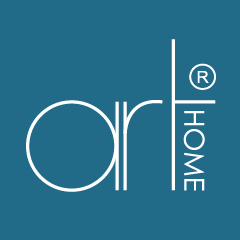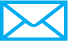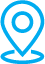1700-822-857arthomefurniture88@gmail.com

## MARBLE

49 - 72 of 161### AIMEE RECTANGULAR MARBLE DINING SET 1+8 (1021T&L (3893)+ S81502)### AIMEE RECTANGULAR MARBLE DINING SET 1+8 (1021T&L(3893) + H2036)### AIMEE RECTANGULAR MARBLE DINING SET 1+8 (1021T&L (3893) + S828)### AIMEE RECTANGULAR MARBLE DINING SET 1+8 (1021T&L(3893) + C608)### PRIMO RECTANGULAR MARBLE DINING SET 1+8 (1021T&L (5609-10)+ S81501)### PRIMO RECTANGULAR MARBLE DINING SET 1+8 (1020T&L (5609-10)+ JOVY-B)### PRIMO RECTANGULAR MARBLE DINING SET 1+8 (1020T&L (5609-10) + S81713)### PRIMO RECTANGULAR MARBLE DINING SET 1+8 (1020T&L (5609-10) + H6035)### PRIMO RECTANGULAR MARBLE DINING SET 1+8 (1020T&L (5609-10)+ S827)### PRIMO RECTANGULAR MARBLE DINING SET 1+8 (1020T&L (5609-10) + C609)### OTTAVIA RECTANGULAR MARBLE DINING SET 1+8 (1020T&L(3817-3) + H6036)### OTTAVIA RECTANGULAR MARBLE DINING SET 1+8 (1020T&L (3817-3) + S81712)### OTTAVIA RECTANGULAR MARBLE DINING SET 1+8 (1020T&L (3817-3) + H2036)### TECHNIC RECTANGULAR MARBLE DINING SET 1+6 (C81501)### TECHNIC RECTANGULAR MARBLE DINING SET 1+6 (H2042)### MATTEO RECTANGULAR MARBLE DINING SET 1+8 (9018T&L (3852) + H2042)### MATTEO RECTANGULAR MARBLE DINING SET 1+8 (9018T&L (3852) +JOVY A)### MATTEO RECTANGULAR MARBLE DINING SET 1+6 (9018T&L (3852) +414191)### MATTEO RECTANGULAR MARBLE DINING SET 1+6 (9028T&L (3852) +S81503)### MATTEO RECTANGULAR MARBLE DINING SET 1+8 (9018T&L (3852) + J4016)### IVANO RECTANGULAR MARBLE DINING SET 1+8 (C609)### IVANO RECTANGULAR MARBLE DINING SET 1+8 (3851-9 + H6037)### IVANO RECTANGULAR MARBLE DINING SET 1+6 (674-1)(J4015)### IVANO RECTANGULAR MARBLE DINING SET 1+6 (C31391)39, 39A, 39B, 41, 41A, 41B, Jalan Pendekar 15, Taman Ungku Tun Aminah, 81300 Skudai, Johor, Malaysia.1700-822-857arthomefurniture88@gmail.com